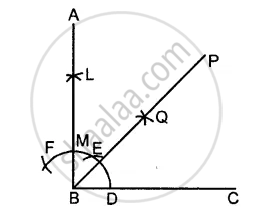# Construct Angle Abc = 90°. Draw Bp, the Bisector of Angle Abc. State, the Measure of Angle Pbc. - Mathematics

Sum

Construct angle ABC = 90°. Draw BP, the bisector of angle ABC. State, the measure of angle PBC.

#### Solution

Draw ∠ABC = 90°Draw bisector of ∠ABC
Then ∠PBC = 1/2 (90°) = 45°

Concept: Construction of an Angle - To Draw the Bisector of a Given Angle
Is there an error in this question or solution?
Chapter 18: Constructions - Exercise 18 (A) [Page 202]

#### APPEARS IN

Selina Concise Mathematics Class 8 ICSE
Chapter 18 Constructions
Exercise 18 (A) | Q 5 | Page 202
Share